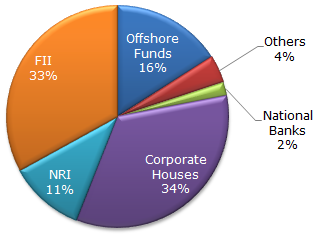# Data Interpretation - Pie Charts - Discussion

The following pie chart shows the amount of subscriptions generated for India Bonds from different categories of investors.

Subscriptions Generated for India Bonds2.

If the investment by NRI's are Rs 4,000 crore, then the investments by corporate houses and FII's together is:

 [A]. 24,000 crore [B]. 24,363 crore [C]. 25,423 crore [D]. 25,643 crore

Explanation:

(67/11) x 4000 = 24 363.6364

 Srikanth said: (Nov 25, 2014) The share of investments by corporate houses and FII's together is 77%. So the answer is (77/11) x 4000 = 28000.

 Kavi said: (May 27, 2015) Anybody please tell me? How comes 11?

 Ravi Pratap Singh said: (Aug 3, 2015) Let total investment is x. Then 11/100*x = 4000. So x = 36363.646. There after 67% of total amount = 67/100*36363.646. = 24363.64.

 Raj said: (Sep 1, 2017) 11% of x = 4000 so wat should be 67% of x. let it be y. 11% (X)=4000 67%(X)=y dividing both 11/67=4000/y y=24363.64.

 Jatinder said: (Oct 4, 2017) Thanks @Raj.

 Sushmitha said: (Apr 27, 2018) How 67% came?

 Peterson said: (Aug 13, 2018) It will be 77%.